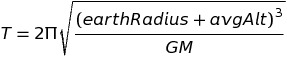Algorithm Map the DebrisRemember to use Read-Search-Ask if you get stuck. Try to pair programand write your own codeProblem Explanation:

The first thing to do is to get familiar with what the program is for by knowing what Orbital period exactly is. You’ve to return a new array that transforms the element’s average altitude into their orbital periods. The parts generally found hard are finding the formula, implementing it and for some people, modifying objects by the key. However, something that is not very clear is the fact that your program has to be able to check for any number of objects in the array; This is what is tested on the second part.Hint: 1

The formula needed is:try to solve the problem nowHint: 2

Use Math.round() to round up to the next whole number as requested. Using Math.ceil() will let you pass the first test but fail the second one.

try to solve the problem nowHint: 3

Find out how to remove and add key to a JavaScript object.

try to solve the problem nowBasic Code Solution:

function orbitalPeriod(arr) {
var GM = 398600.4418;
var earthRadius = 6367.4447;
var a = 2 * Math.PI;
var newArr = [];
var getOrbPeriod = function(obj) {
var c = Math.pow(earthRadius + obj.avgAlt, 3);
var b = Math.sqrt(c / GM);
var orbPeriod = Math.round(a * b);
delete obj.avgAlt;
obj.orbitalPeriod = orbPeriod;
return obj;
};

for (var elem in arr) {
newArr.push(getOrbPeriod(arr[elem]));
}

return newArr;
}

// test here
orbitalPeriod([{name : "sputnik", avgAlt : 35873.5553}]);Run Code

Code Explanation:

• GM and earthRadius are both given to us.
• To make the code easier to edit and read, each part of the equation is written separately.
• Create newArr to store the orbPeriod's.
• a is 2 times pi. The part that is a constant is on the global scope while the rest is part of a function.
• Create a function, gerOrbPeriod() that will do the required work for any amount of objects.
• c is (earthRadius + avgAlt) to the cube.
• b is the square root of c divided by GM.
• Create orbPeriod to store the product of a and b, with the Math.round() function applied to round up to the next whole number.
• Then we delete the key avgAlt, and add the new key and its value.Intermediate Code Solution:

function orbitalPeriod(arr) {
var GM = 398600.4418;
var earthRadius = 6367.4447;

//Looping through each key in arr object
for(var prop in arr) {
//Rounding off the orbital period value
var orbitalPer = Math.round(2 * Math.PI * Math.sqrt(Math.pow(arr[prop].avgAlt + earthRadius, 3) / GM));
//deleting the avgAlt property
delete arr[prop].avgAlt;
arr[prop].orbitalPeriod = orbitalPer;
}

return arr;
}

// test here
orbitalPeriod([{name : "sputnik", avgAlt : 35873.5553}]);Run Code

Code Explanation:

• GM and earthRadius are both given to us.
• A for..in loop is used to iterate through each value in given array arr.
• orbitalPer holds the value of orbital period for each iteration calculated using the formula.
• The key avgAlt is deleted, and orbitalPer found is assigned in arr.Advanced Code Solution:

function orbitalPeriod(arr) {
var GM = 398600.4418;
var earthRadius = 6367.4447;

// Loop through each item in the array arr
arr.forEach(function(item) {
// Calculate the Orbital period value
var tmp = Math.round(2 * Math.PI * Math.sqrt(Math.pow(earthRadius + item.avgAlt, 3) / GM));
//Delete the avgAlt property
delete item.avgAlt;
item.orbitalPeriod = tmp;
});
return arr;
}

// test here
orbitalPeriod([{name : "sputnik", avgAlt : 35873.5553}]);Run Code

Code Explanation:

• GM and earthRadius are both given to us.
• The forEach() method is used to execute the function once per element (item) in arr.
• tmp holds the value of orbital period for each element calculated using the formula.
• The key avgAlt is deleted, and orbital period (tmp) found is assigned to the key orbitalPeriod.Credits:

If you found this page useful, you may say thanks to the contributors by copying and pasting the following line in the main chat:

Thanks @Rafase282 @coded9 @anuragaryan for your help with Algorithm: Map the DebrisNOTES FOR CONTRIBUTIONS:

•DO NOT add solutions that are similar to any existing solutions. If you think it is similar but better, then try to merge (or replace) the existing similar solution.
• Add an explanation of your solution.
• Categorize the solution in one of the following categories — Basic, Intermediate and Advanced.• Please add your username only if you have added any relevant main contents. (DO NOT remove any existing usernames)

SeeWiki Challenge Solution Template for reference.